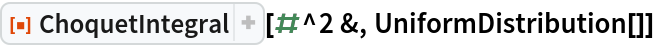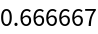Function Repository Resource:

# ChoquetIntegral

Compute numerically the Choquet integral of a function with respect to a probability distribution

Contributed by: Carl Woll
 ResourceFunction["ChoquetIntegral"][f, dist] gives the Choquet integral of f with respect to the probability distribution dist.

## Details and Options

A Choquet integral is an integral sometimes used to denote the expected utility of a function.
The function f should be a pure function or a symbol with DownValues.

## Examples

### Basic Examples (1)

The Choquet integral of f(x)=x2 with respect to a uniform distribution:

 In:=Out=Carl Woll

## Version History

• 1.0.0 – 16 October 2019